output.to from Sideway
Draft for Information Only

# Content

`Magnetic Torque Motor  Equilibrium  Commutator  Force on a Magnetic Dipole Generator Source and Reference`

# Magnetic TorqueA magnetic field exerts a torque on a loop of wire that carries current. The magnetic torque causes the loop of wire to spin around a fixed axis.

## Motor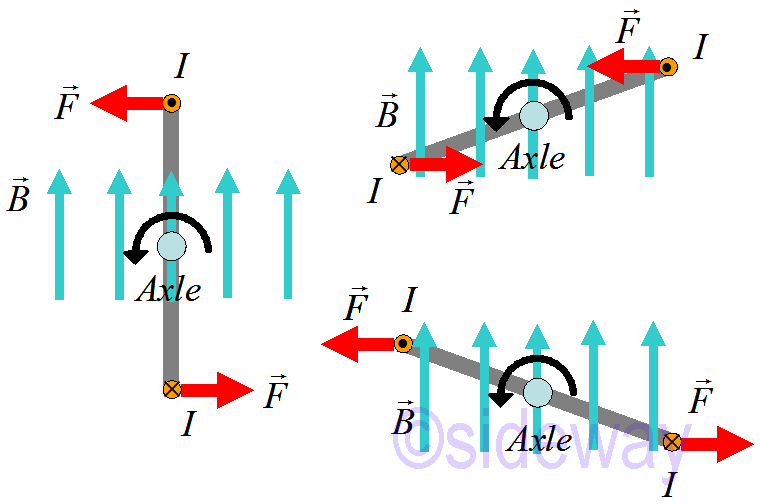In the above orientations, the loop of wire twists counterclockwise.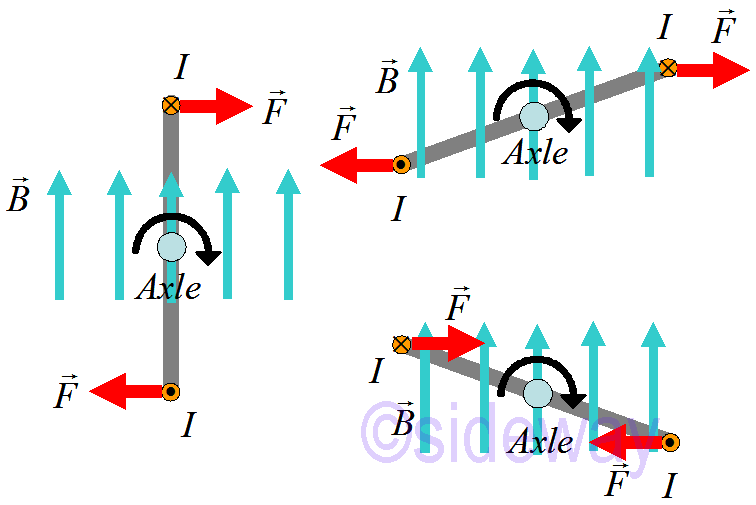In the above orientations, the loop of wire twists clockwise.

### Equilibrium

When the loop of wire is perpendicular to the magnetic field, the motion of wire loop may be in unstable equilibrium and a slight deviation does not return to the equilibrium state as followingBesides, the loop of wire is perpendicular to the magnetic field, the motion of wire loop may be in stable equilibrium and a slight deviation returns to the equilibrium state as following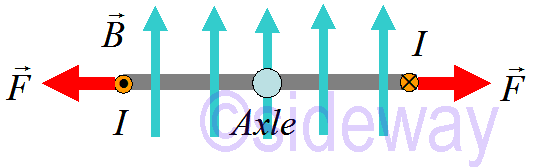### Commutator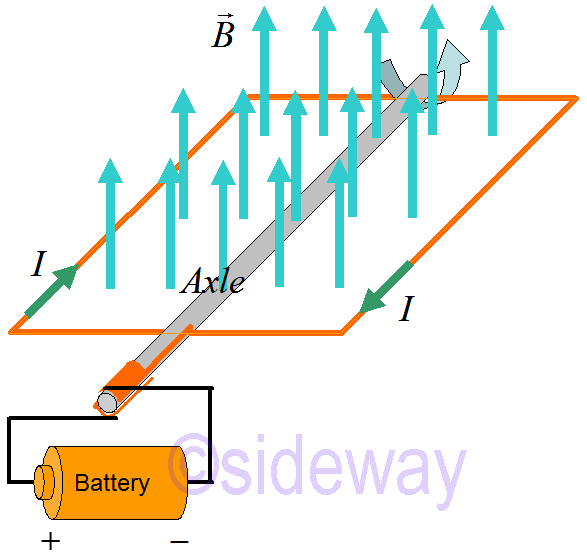In order to to prevent the stable equilibrium state of the loop of wire and to maintain the loop of wire twists counterclockwise, a commutator is used.

### Force on a Magnetic Dipole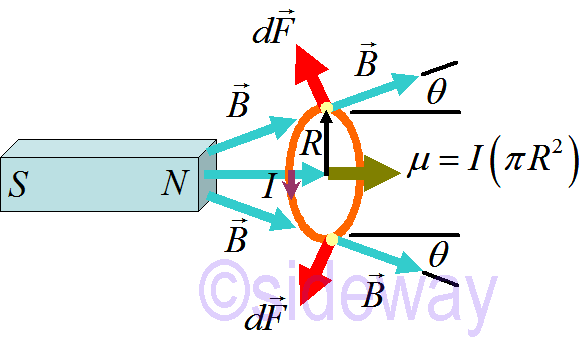Magnetic force 𝑑𝐹=𝐼𝑑𝑙×𝐵, is not point to the left. Therefore using a sneaky way to find the net force to the left 𝐹magnet by equating the magnetic force pulling the coil to the left due to moving the coil to the right by an external force.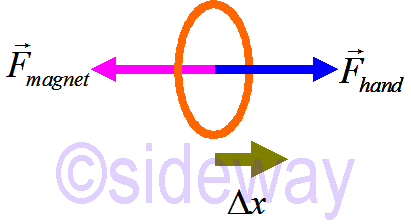```𝑊=∆𝑈, 𝑈=−𝜇⋅𝐵 ∆𝑈=𝑊by hand=𝐹by hand∆𝑥=−𝐹magnet∆𝑥 𝐹magnet=−∆𝑈∆𝑥→𝐹magnet=−𝑑𝑈𝑑𝑥 if 𝜇 is constant (typical case), then 𝐹magnet=𝜇𝑑𝐵𝑑𝑥 if 𝐵 is constant, then net 𝐹magnet=0 ```

## Generator

Generator converts mechanical energy into electrical energy.## Source and Reference

ID: 200101802 Last Updated: 1/18/2020 Revision: 0Home 5

Management

HBR 3

Information

Recreation

Culture

Chinese 1097

English 337

Computer

Hardware 156

Software

Application 207

Latex 35

Manim 203

Numeric 19

Programming

Web 285

Unicode 504

HTML 65

CSS 65SVG 9

ASP.NET 270OS 422

Python 66Knowledge

Mathematics

Algebra 84

Geometry 32

Calculus 67

Engineering

Mechanical

Rigid Bodies

Statics 92

Dynamics 37

Control

Natural Sciences

Electric 27